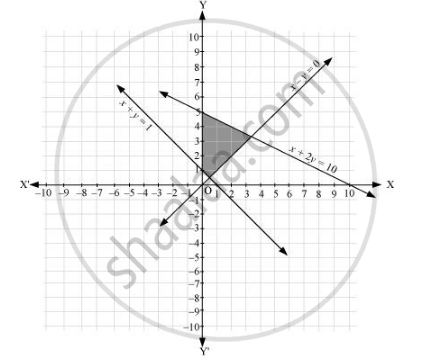CBSE (Arts) Class 11CBSE
Share

# Solve the Following System of Inequalities Graphically: X + 2y ≤ 10, X + Y ≥ 1, X – Y ≤ 0, X ≥ 0, Y ≥ 0 - CBSE (Arts) Class 11 - Mathematics

ConceptSolution of System of Linear Inequalities in Two Variables

#### Question

Solve the following system of inequalities graphically: x + 2y ≤ 10, x + y ≥ 1, x – y ≤ 0, x ≥ 0, y ≥ 0

#### Solution

x + 2y ≤ 10 … (1)

x + y ≥ 1 … (2)

x – y ≤ 0 … (3)

The graph of the lines, x + 2y = 10, x + y = 1, and x – y = 0, are drawn in the figure below.

Inequality (1) represents the region below the line, x + 2y = 10 (including the line x + 2y = 10). Inequality (2) represents the region above the line, x + y = 1 (including the line x + y = 1). Inequality (3) represents the region above the line, x – y = 0 (including the line x – y = 0).

Since x ≥ 0 and y ≥ 0, every point in the common shaded region in the first quadrant including the points on the respective lines and the axes represents the solution of the given system of linear inequalities.Is there an error in this question or solution?

#### APPEARS IN

NCERT Solution for Mathematics Textbook for Class 11 (2018 to Current)
Chapter 6: Linear Inequalities
Q: 15 | Page no. 129

#### Video TutorialsVIEW ALL 

Solution Solve the Following System of Inequalities Graphically: X + 2y ≤ 10, X + Y ≥ 1, X – Y ≤ 0, X ≥ 0, Y ≥ 0 Concept: Solution of System of Linear Inequalities in Two Variables.
S